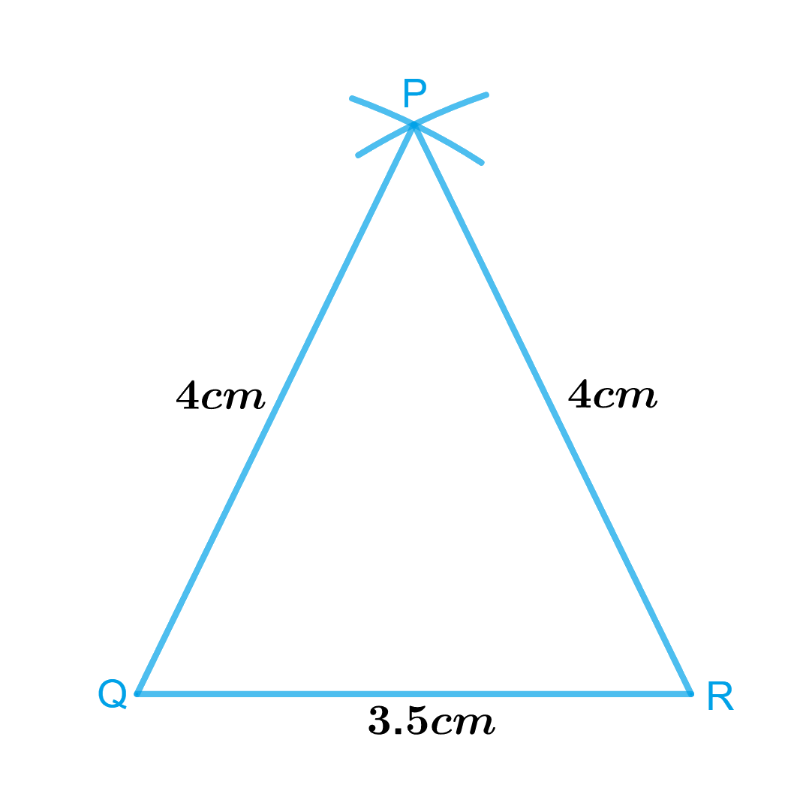# Ex.10.2 Q3 Practical Geometry Solution - NCERT Maths Class 7

Go back to  'Ex.10.2'

Draw  $$ΔPQR$$ with $$PQ = 4\,\rm{cm}$$, $$QR = 3.5\,\rm{cm}$$ and $$PR = 4\,\rm{cm}$$. What type of triangle is this?

What is known?

Lengths of sides of a triangle are $$PQ = 4\,\rm{cm}$$, $$QR = 3.5\,\rm{cm}$$ and $$PR = 4\,\rm{cm}$$.

To construct:

$$ΔPQR$$ with $$PQ = 4\,\rm{cm}$$, $$QR = 3.5\,\rm{cm}$$ and $$PR = 4\,\rm{cm}$$.

Reasoning:

To construct a $$ΔPQR$$ with $$PQ = 4 \,\rm{cm,}\, QR = 3.5\,\rm{ cm}$$ and $$PR = 4 \,\rm{cm}$$, follow the steps given below.

Steps:Steps of construction

1. Draw a line segment $$QR$$ of length $$3.5\, \rm{cm}.$$
2. From $$Q$$, point $$P$$ is at a distance of $$4\,\rm{cm}$$. So, with $$Q$$ as centre, draw an arc of radius $$4\,\rm{cm}$$ (now $$P$$ will be somewhere on this arc & our job is to find where exactly $$P$$ is).
3. From $$R$$, point $$P$$ is at a distance of $$4\,\rm{cm}$$. So, with $$R$$ as centre, draw an arc of radius $$4\,\rm{cm}$$ (now $$P$$ will be somewhere on this arc, we have to fix it).
4. $$P$$ has to be on both the arcs drawn. So, it is the point of intersetion of arcs. Mark the point of intersection of arcs as $$P$$ join $$PQ$$ and $$PR.$$

Thus, $$PQR$$ is the required triangle.

$$\Delta PQR$$ is an isosceles triangle as two of the sides are equal.